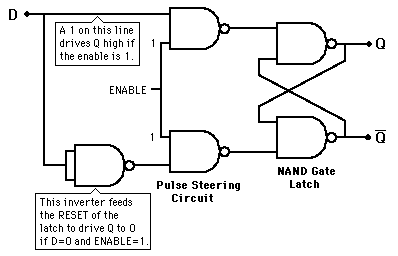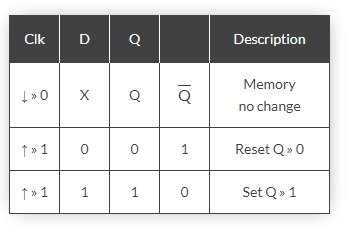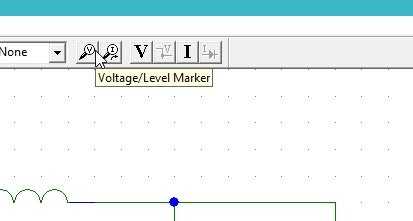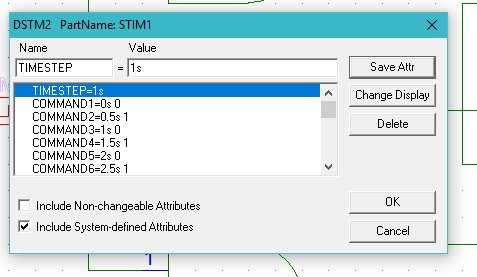# D Flip Flops simulation using PSpice : tutorial 12

In this tutorial I will explain you the working of a simple digital system known as a Flip flop. At the start a brief and concise introduction to flip flops specifically a simplest D flip flop is provided with the explanation of the output they will show. Flip flop is another form of latches which is used to store the memory in two or more states. After that the circuits is simulated using PSPICE and the result are compared with the theoretical discussion provided (which should be same). At the end of the tutorial you are provided with an exercise to do it by yourself, and in the next tutorials I will assume that you have done those exercises and I will not explain the concept regarding them.

### Introduction to D flip flop

D flip flop also known as a Master-Slave flip flop is a modified form of a set reset (SR) flip flop. Only the reset side of the flip flop is passed through an inverter as shown in the figure below,Figure 1: D flip flop

The Q output of the D or Data flip flop will be the same as the input if the enable is 1 and Q will show the previous input if the enable is 0. However, the Q’ output is the inverted form of Q as shown in the truth table below,Figure 2: Truth table

### simulation of D Flip Flip in PSpice

Figure 3: Opening schematic

Figure 4: Saving schematic

Figure 5: Getting new part

Figure 6: Placing NAND gate

Figure 7: Placing digital supply

Figure 8: Placed components

Figure 9: Drawing wire

Figure 10: Complete circuit diagram

Figure 11: Labeling

Figure 12: Labeled circuit diagrams

• We can also change the name of the device i.e. in this case we have 2 identical sources however each of these have separate purpose. One of the sources are used as inputs of the flip flop and the other is used for the enable of the flip flop. If you want to display the voltage at a specific node of the circuit diagram you can do this by placing a voltage marker at that node. On the top of the schematic window, click on the Voltage/Level Marker button as shown in the figure below,Figure 13: Voltage marker

Figure 14: Placed voltage marker

Figure 15: Input attributes

• The commands in the attributes window represents the value of the input at the corresponding time in the command before the space. The COMMAND1 = 0s 0 shows that at time 0s the value of the input dstm is 0 and the COMMAND2 = 1s 1 shows that the value will update to 1 at time 1 second. Similar is the case with COMMAND5 = 4s 0 that at 4 seconds the value of the input will be 0.
• Next step is to set the attributes of the input digital supply. Double click on the dstm2 which is used as the enable source of the flip flop supply you connected in the circuit previously and set the input commands of the digital input system but these commands may or may not be same to the commands of input 1 as shown in the figure below,Figure 16: Enable attributes

Figure 17: Simulation setup

Figure 18: Transient properties

Figure 19: Simulation

Figure 20: Output

The output of the schematic is in accordance with the output discussed in the introduction part. The enable will control the output of the flip flop. When the enable is one the Q output of the flip flop will give the input as it is and the Q’ output will give the inverted input and when the enable is zero the Q output will give the previous input value at the output and Q’ output will give the previous inverted input at the output. At the start the red line at the outputs shows that there was no previous input i.e. the input was not assigned any value in the previous state. As the enable is zero in the start the output will be the previous input state which is undefined.

Exercise:

• Design a JK flip flop using the criteria discussed in this tutorial.

<< Previous tutorial                                                                     Next tutorial>>